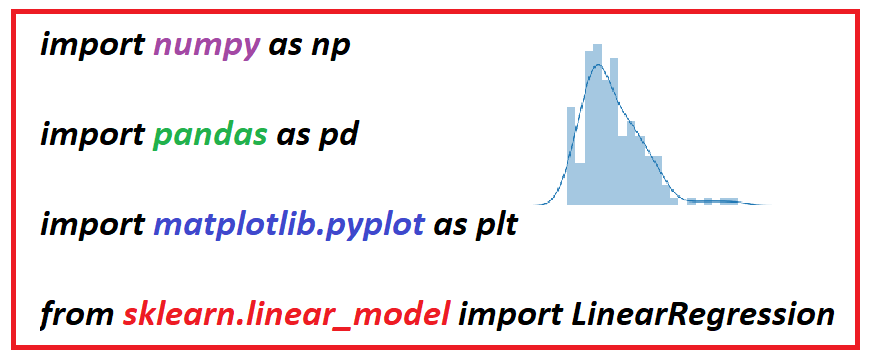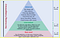# 4 Python Libraries for Basic Data Science

## For levels 1 and 2 data science, mastery of pandas, numpy, matplotlib, and scikit-learn is essentialImage by Benjamin O. Tayo

# Introduction

For levels 1 and 2 data science, mastery of pandas, numpy, matplotlib, and scikit-learn libraries is essential. If you master these 4 packages, then you should be able to perform level 1 and 2 tasks using Python, as outline below.Image by Benjamin O. Tayo

# 1. Basic Level

At level one, a data science aspirant should be able to work with datasets generally presented in comma-separated values (CSV) file format. They should have competency in data basics; data visualization; and linear regression.

Be able to manipulate, clean, structure, scale, and engineer data. They should be skilled in using pandas and numpy libraries. Should have the following competencies:

• Know how to import and export data stored in CSV file format
• Be able to clean, wrangle, and organize data for further analysis or model building
• Be able to deal with missing values in a dataset
• Understand and be able to apply data imputation techniques such as mean or median imputation
• Be able to handle categorical data
• Know how to partition a dataset into training and testing sets
• Be able to scale data using scaling techniques such as normalization and standardization
• Be able to compress data via dimensionality reduction techniques such as principal component analysis (PCA)

Be able to understand the essential components of good data visualization. Be able to use data visualization tools including Python’s matplotlib and seaborn packages. Should understand the essential components of good data visualization:

• Data Component: An important first step in deciding how to visualize data is to know what type of data it is, e.g., categorical data, discrete data, continuous data, time-series data, etc.
• Geometric Component: Here is where you decide what kind of visualization is suitable for your data, e.g., scatter plot, line graphs, bar plots, histograms, Q-Q plots, smooth densities, boxplots, pair plots, heatmaps, etc.
• Mapping Component: Here, you need to decide what variable to use as your x-variable and what to use as your y-variable. This is important especially when your dataset is multi-dimensional with several features.
• Scale Component: Here, you decide what kind of scales to use, e.g., linear scale, log scale, etc.
• Labels Component: This includes things like axes labels, titles, legends, font size to use, etc.
• Ethical Component: Here, you want to make sure your visualization tells the true story. You need to be aware of your actions when cleaning, summarizing, manipulating, and producing a data visualization and ensure you aren’t using your visualization to mislead or manipulate your audience.

Be familiar with linear regression and other advanced regression methods. Be competent in using packages such as scikit-learn and caret for linear regression model building. Have the following competencies:

• Be able to perform simple regression analysis using numpy
• Be able to perform multiple regression analysis with scikit-learn
• Understand regularized regression methods such as Lasso, Ridge, and Elastic Net
• Understand other non-parametric regression methods such as KNeighbors regression (KNR), and Support Vector Regression (SVR)
• Understand various metrics for evaluating a regression model such as MSE (mean square error), MAE (mean absolute error), and R2 score
• Be able to compare different regression models

# 2. Intermediate Level

In addition to skills and competencies in level I, should have competencies in the following:

Be familiar with binary classification algorithm such as:

• Perceptron classifier
• Logistic Regression classifier
• Support Vector Machines (SVM)
• Be able to solve nonlinear classification problems using kernel SVM
• Decision tree classifier
• K-nearest classifier
• Naive Bayes classifier
• Understand several metrics for accessing the quality of a classification algorithm such as accuracy, precision, sensitivity, specificity, recall, f-l score, confusion matrix, ROC curve.
• Be able to use scikit-learn for model building
• Be able to combine transformers and estimators in a pipeline
• Be able to use k-fold cross-validation to assess model performance
• Know how to debug classification algorithms with learning and validation curves
• Be able to diagnose bias and variance problems with learning curves
• Capable of addressing overfitting and underfitting with validation curves
• Know how to fine-tune machine learning models via grid search
• Understand how to tune hyperparameters via grid search
• Be able to read and interpret a confusion matrix
• Be able to plot and interpret a receiver operating characteristic (ROC) curve

All these tasks can be performed using scikit-learn.

• Be able to use the ensemble method with different classifiers
• Be able to combine different algorithms for classification
• Know how to evaluate and tune the ensemble classifier

All these tasks can be performed using scikit-learn.

# How to Learn the Essential Python Libraries

The Python documentation is a very useful tool for learning about Python libraries. The documentation can be accessed using a question sign before a given command.

• For example, to find out more about the pd.read_csv() method, we could use the code below.
`import pandas as pd?pd.read_csv`

This would take you to the Python documentation where you can learn more about the pd.read_csv() method, including its various adjustable parameters.

• To find out more about the logistic regression classifier, one could use the following code to access the Python documentation file:
`from sklearn.linear_model import LogisticRegression?LogisticRegression`

This would open documentation that contains more information about the LogisticRegression classifier including a detailed explanation of all parameters and attributes.

It is important that when building a model using the scikit-learn library, you understand the various adjustable parameters for each estimator. Using default parameters will not always produce the optimal results. For example, logistic regression has the following parameters:

`LogisticRegression(penalty ='l2',dual=False, tol=0.0001, C=1.0,                       fit_intercept=True, intercept_scaling=1,                    class_weight=None, random_state=None,                    solver='liblinear', max_iter=100,                    multi_class='ovr', verbose=0,                   warm_start=False, n_jobs=1)`

For logistic regression, the C parameter (regularization parameter) is very crucial, thus instead of using the default value, it’s good to tune your model performance for different C values before choosing the C value that yields optimal performance.

# Summary and Conclusion

In summary, we’ve discussed 4 essential Python libraries that can get you through level 1 and 2 in your data science journey. The most effective way to learn about these libraries is by using the Python Documentation.

# Additional data science/machine learning resources

Timeline for Data Science Competence

Data Science Curriculum

Essential Maths Skills for Machine Learning

3 Best Data Science MOOC Specializations

A Data Science Portfolio is More Valuable than a Resume

Physicist, Data Science Educator, Writer. Interests: Data Science, Machine Learning, AI, Python & R, Personal Finance Analytics, Materials Sciences, Biophysics

Physicist, Data Science Educator, Writer. Interests: Data Science, Machine Learning, AI, Python & R, Personal Finance Analytics, Materials Sciences, Biophysics